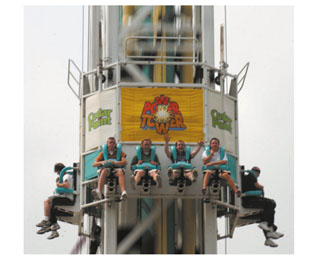# Problem: Riders on the Power Tower are launched skyward with an acceleration of 4g, after which they experience a period of free fall. (Figure 1)Part AWhat is a 60 kg rider's apparent weight during the launch?Express your answer to two significant figures and include the appropriate units.w =Part BWhat is a 60 kg rider's apparent weight during the period of free fall?Express your answer to two significant figures and include the appropriate units.w =

###### FREE Expert Solution

Newton's second law:

$\overline{){\mathbf{\Sigma }}{\mathbf{F}}{\mathbf{=}}{\mathbf{m}}{\mathbf{a}}}$

Part A:

N - mg = ma

N = m(a + g)

N = m (4g + g) = 5mg

81% (36 ratings)###### Problem Details

Riders on the Power Tower are launched skyward with an acceleration of 4g, after which they experience a period of free fall. (Figure 1)Part A

What is a 60 kg rider's apparent weight during the launch?

Express your answer to two significant figures and include the appropriate units.

w =

Part B

What is a 60 kg rider's apparent weight during the period of free fall?

Express your answer to two significant figures and include the appropriate units.

w =